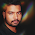close

# JavaScript Array Deobfuscation Automation TechniqueDeobfuscation means, decoding JavaScript code and converting it from unreadable to readable.

Obfuscation is a technique to protect your computer code from reuse.

There are different methods or ways to obfuscate your computer codes. Especially JavaScript code and one of them is an array Obfuscation method.

You can easily deobfuscate your JavaScript code using online JavaScript unpacker or deobfuscator tool which I have shared with you.

Sometimes it becomes difficult to deobfuscate JavaScript code especially the arrays.

If you don't know about arrays let me tell you that. JavaScript arrays look like this.

``var array = ['HTML', 'CSS', 'JavaScript']``

This is the array and to call any value from the array we use array name with the index to get the specified value. The array index starts from 0. Let me show you the example of calling any entry from the array with its index.

``array``

It will return the value "HTML", The first Key value.

That was about the JavaScript arrays and now let me tell you what is the purpose of this article.

I wrote this article to tell you how you can put the array values to its calls and remove the array.

If you are unable to understand the above line let me show you the preview.

## Before manipulation your code looks like:

``````var array = ['HTML', 'CSS', 'JavaScript'];
var a = array;
var b = array;
var c = array;``````

## After Array Deobfuscation your code looks like this:

``````var a = "HTML";
var b = "CSS";
var c = "JavaScript";``````

Use this code to manipulate your arrays and replace array calls to the values provided in the array.
``````<textarea id='returnCode' style='height:40%;width:100%'></textarea>
<script>
var array = ["lazyify", "fn"];
function automate(){
for (i = 0; i < array.length; i++) {
var ptrn = /("|')(.*?)(\1)(,|])/g;
var arrayIndex = i;
var arrayVal = array[arrayIndex];
var regex = new RegExp("array\\[" + arrayIndex + "\\]", "g");
\$('#returnCode').val(\$('#returnCode').val().replace(regex, '"'+arrayVal+'"'));
}
}
</script><button onclick='automate()'>Automate</button>``````

This code will help you when array values are called randomly by a function.
``````function automate(){
for (i = 0; i < array.length; i++) {
var arrayVal = getArrayValue(i);// array[arrayIndex];
\$('#returnCode').val(\$('#returnCode').val().replace("getArrayValue\(" + i + "\)", '"'+arrayVal+'"'));
}
}``````

This regex will also be useful. How? I don't Know :).
``document.write\('.replace\("\$1", '+\$1+'\)'\);``

## Usage of the above code:

In the above code you simply have to replace the array and array name everywhere it is used in the code and paste your code in the text area and all the array calls will be transformed into strings.

Deobfuscation is also called reverse engineering.

## Why there is a need for this code?

Using this code you can replace array calls to the values that are called using array index automatically. Without it, you have to replace array calls with the values manually one by one.

## Conclusion:

1.Can you please give a demo

1.What Do You mean by demo?

2.How to deobfuscate an Array

1.3.thanks bro..

1.